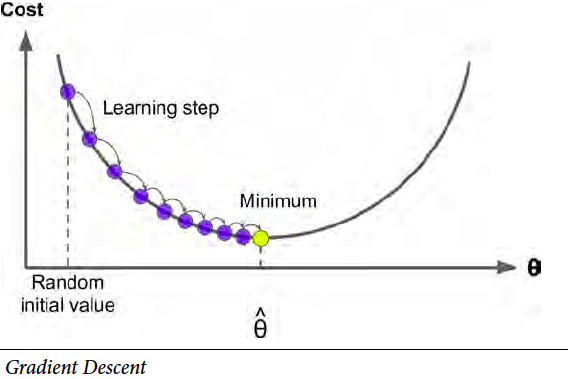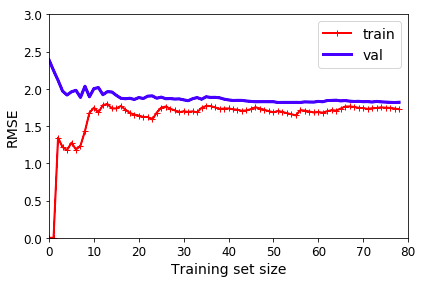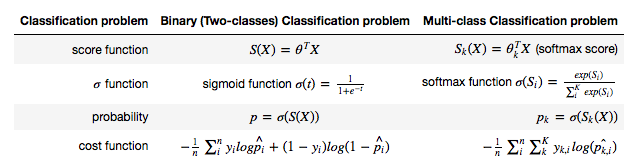# Summary of Chapter 4, Regression 第四章总结， 回归问题

How much is a 1000 square feet house? How many customers can I expect tomorrow? Let’s take a look at machine learning approach on regression problem. 百平米房子多少钱？明天能来多少顾客？让我们看一下机器学习如何解决回归问题。

In this chapter summary, I will show how linear and logistic regression works. Linear algebra behind the model is not the focus.

Here is the structure of this summary. 这是本总结的结构

1. Linear regression 线性回归
2. Regularization
2. Logistic regression 逻辑回归
1. Logit Regression
2. Softmax Regression

### Linear Regression 线性回归

Simple linear regression is one of the most basic machine learning models. It has been well studied from all aspects by statisticians, for example, closed-form solution, ANOVA test on lack of fit, confidence interval, confidence band, prediction interval, residual variance check, p-value on each parameter, etc. While statisticians care a lot about fitting and confidence, machine learners don’t seem to really care that much. Instead, cross-validation, hyper-parameter tuning, memory and time complexity, underfitting and overfitting, learning rate are more of interest to machine learners. So one can get some ideas on the difference between statistics and machine learning, on both their approaches and goals.

When you have more than one feature, use Multivariant linear regression. If the data is more complex than a straight line or hyperplane, use Polynomial linear regression.

It is well-known that there is closed-form solution for simple linear regression. However, it does not really fit large datasets. This is because it needs to calculate the reverse operation of a matrix of size NxN, where N stands for a number of instances. The time complexity of closed-form solution is about $O(m^{2.4})\sim O(m^3)$ for number of features $m$, and $O(n)$ for number of instance $n$. What’s more, it requires an adequate amount of memory. The closed-form solution could easily blow off the memory space for large datasets.

To handle large datasets, gradient descent is used. The idea is to improve the parameters by a small amount every step before eventually reaching optimal values. It is very “computer science”. The approach is called Batch Gradient Descent.

However, it is still very memory-expensive to dump the whole dataset into matrix calculation. So a derived version, Stochastic Gradient Descent (SGD), is more commonly used where only one instance data is used at a time. The word stochastic refers to the fact that the order of data feed is stochastic. This approach is much faster than Batch gradient descent with some small drawbacks (not discussed here).

Just to make the picture complete, I need to mention a combined version of the two approaches mentioned above is called Mini-batch Gradient Descent.It worth mentioning the learning rate hyper-parameter. If the learning rate is too large, you end up jumping around the optimal solution instead of hitting it. If the learning rate is too small, the training can take a long time. Also, small learning rate may lead you to the trap of local minimal.

# To support both python 2 and python 3
from __future__ import division, print_function, unicode_literals

import numpy as np
import pandas as pd
import matplotlib.pyplot as plt
plt.rcParams['axes.labelsize'] = 14
plt.rcParams['xtick.labelsize'] = 12
plt.rcParams['ytick.labelsize'] = 12
%matplotlib inline

X = 2 * np.random.rand(100, 1)
y = 4 + 3 * X + np.random.randn(100, 1)
from sklearn.linear_model import SGDRegressor
sgd_reg = SGDRegressor(max_iter=50, penalty=None, eta0=0.1)
sgd_reg.fit(X, y.ravel())
sgd_reg.intercept_, sgd_reg.coef_

(array([3.80549194]), array([3.28373567]))


#### Regularization 正则

The performance of the model can be evaluated with validation set. For example, errors plot for both training set and validation set gives a very good idea of how well the model is. Here is a figure of error vs training size set.

from sklearn.metrics import mean_squared_error
from sklearn.model_selection import train_test_split
def plot_learning_curves(model, X, y):
X_train, X_val, y_train, y_val = train_test_split(X, y, test_size=0.2)
train_errors, val_errors = [], []
for m in range(1, len(X_train)):
model.fit(X_train[:m], y_train[:m])
y_train_predict = model.predict(X_train[:m])
y_val_predict = model.predict(X_val)
train_errors.append(mean_squared_error(y_train_predict, y_train[:m]))
val_errors.append(mean_squared_error(y_val_predict, y_val))
plt.plot(np.sqrt(train_errors), "r-+", linewidth=2, label="train")
plt.plot(np.sqrt(val_errors), "b-", linewidth=3, label="val")

from sklearn.linear_model import LinearRegression
lin_reg = LinearRegression()
plot_learning_curves(lin_reg, X, y)If the gap between training and validation set is very big, the model is probably overfitting. To reduce overfitting, regularization is added to the model. Three approaches are commonly used.

• Ridge
• Lasso
• Elastic Net (a combination of Ridge of Lasso)

• Ridge
• Lasso
• Elastic Net (a combination of Ridge of Lasso)

### Logistic Regression 逻辑回归

Logistic regression is one of the basic classification models. It is usually discussed right after linear regression in many machine learning materials since their model structures shares a lot in common.

Recall that output from linear regression is in the range of $(-\infty,\infty)$. The main idea of logistic regression is to convert the score function result from the range of $(-\infty,\infty)$ to $(0,1)$, which is the desired range for probability. For binary classification problems, use Logit regression as the conversion function. For multi-class classification problems, use Softmax regression. Correspondingly, the cost function has also changed. It is the mean of log probability of the prediction result.

A comparison table says 100 times more than words.This article is part of a series of summaries on the book Hands-On Machine Learning with Scikit-Learn and TensorFlow. The summaries are meant to explain machine learning concepts and ideas, instead of covering the maths and models. 本文是《Hands-On Machine Learning with Scikit-Learn and TensorFlow》这本书的总结随笔系列的一部分。总结旨在解释机器学习的观念和想法，而不是数学和模型

Written on March 31, 2018